• 2
•
•
•
•
•
•
•
2
Shares

# TRUE DISCOUNT AND BANKER’S DISCOUNT TRICKS IN HINDI## TRUE DISCOUNT

1). True Discount = Amount – Present worth
2). If the rate of interest is r% p.a., time t and present worth (PW), then the actual discount is-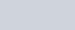3). If r% and money payable after time t is A, then the present worth is-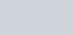4). If the money payable on A is given at r% and time t, then the true discount is-5). If after a certain period of time, at a fixed annual rate, the true discount (TD) on the money due and the simple interest (SI) for the same time and rate, then the money payable A is-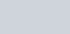6). If t time after, the true discount (TD) and simple interest (SI) on the amount due at r% per annum, then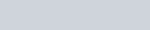7). Money A’s present worth (PW) due after t years at r% compounded is-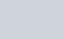And the true discount is-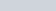## BANKER’S DISCOUNT

1). Banker’s discount = interest on the bill for the remaining time (unexpired time) =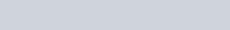2). Banker’s profit = Banker’s discount – true discount
3). If the marked price of the bill is A, time t and rate is r%, then the Banker’s discount-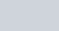4). At time t and rate r% on a bill of marked price A –
*. Banker’s profit =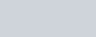*. Banker’s profit =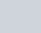5). If the true discount on a bill payable after time t is TD, and the rate of interest per annum is r%, then the banker’s profit is-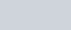6). If the marked price on the bill is A and the true discount is TD, then the banker’s discount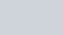7). If the banker’s profit on a bill payable after time t at r% simple interest is BP, then the present worth (PW) is-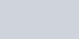8). If the banker’s profit on a bill is BP and the present worth is PW, then the true discount (TD)-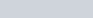9). If banker’s discount (BD) and true discount (TD) is given on a bill, then the amount of bill A is-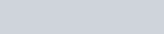10). If the banker’s discount on a sum a for a certain time at the rate of r% interest, and the true discount on a sum of b for the same rate and time is equal, then the value of time-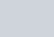11). If the rate of interest and time on a bill are numerically equal, and the true discount is n times the banker’s discount, then the rate of interest or time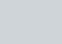### Abbreviations to be noted

TD = True Discount
PW = Present worth
A = Amount
SI = simple interest
CI = Compound interest
BD = Banker’s Discount
BP = Bankers Profit